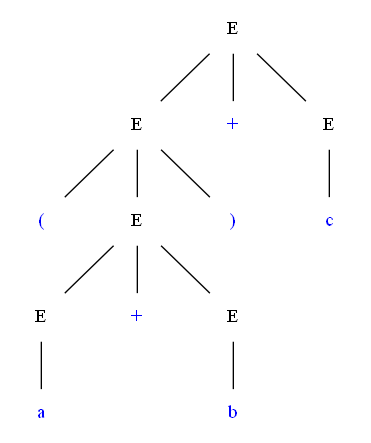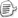E → idE → E + EE → ( E )

E  =>  E + E  =>  (E) + E  =>  (E + E) + E  =>  (a + E) + E  =>  (a + b) + E  =>  (a + b) + c

E  =>  E + E  =>  E + c  =>  (E) + c  =>  (E + E) + c  =>  (E + b) + c  =>  (a + b) + cE → idE → E + EE → E * EE → ( E )

 F → idF → ( E )T → T * FT → FE → E + T E → Tposted on 2011-06-18 20:27  装配脑袋  阅读(22103)  评论(20编辑  收藏  举报